Open in App
Not now

# Code Converters – BCD(8421) to/from Excess-3

• Difficulty Level : Medium
• Last Updated : 05 Jan, 2022

Prerequisite – Number System and base conversions

Excess-3 binary code is an unweighted self-complementary BCD code.
Self-Complementary property means that the 1’s complement of an excess-3 number is the excess-3 code of the 9’s complement of the corresponding decimal number. This property is useful since a decimal number can be nines’ complemented (for subtraction) as easily as a binary number can be ones’ complemented; just by inverting all bits.
For example, the excess-3 code for 3(0011) is 0110, and to find the excess-3 code of the complement of 3, we just need to find the 1’s complement of 0110 -> 1001, which is also the excess-3 code for the 9’s complement of 3 -> (9-3) = 6.

### Converting BCD(8421) to Excess-3 –

As is clear by the name, a BCD digit can be converted to its corresponding Excess-3 code by simply adding 3 to it. Since we have only 10 digits(0 to 9) in decimal, we don’t care about the rest and marked them with a cross( X ).
Letbe the bits representing the binary numbers, whereis the LSB andis the MSB, and
Letbe the bits representing the gray code of the binary numbers, whereis the LSB andis the MSB.
The truth table for the conversion is given below. The X’s mark is don’t care condition.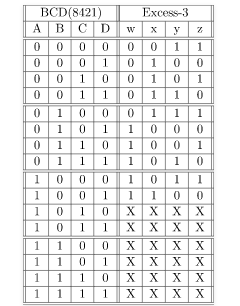To find the corresponding digital circuit, we will use the K-Map technique for each of the Excess-3 code bits as output with all of the bits of the BCD number as input.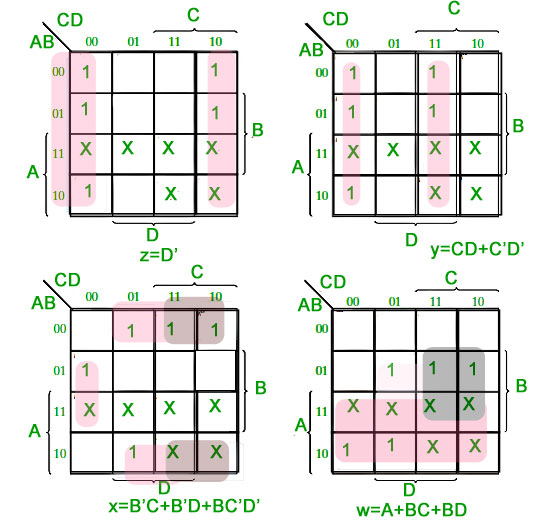Corresponding minimized Boolean expressions for Excess-3 code bits –The corresponding digital circuit-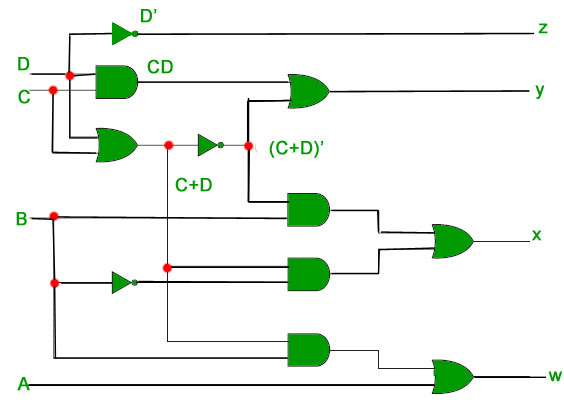### Converting Excess-3 to BCD(8421) –

Excess-3 code can be converted back to BCD in the same manner.
Letbe the bits representing the binary numbers, whereis the LSB andis the MSB, and
Letbe the bits representing the gray code of the binary numbers, whereis the LSB andis the MSB.
The truth table for the conversion is given below. The X’s mark is don’t care condition.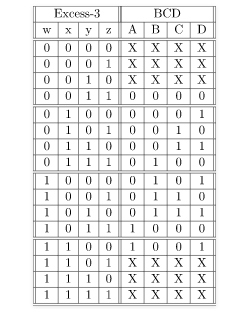K-Map for D-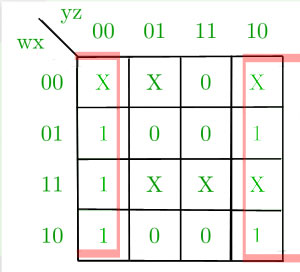K-Map for C-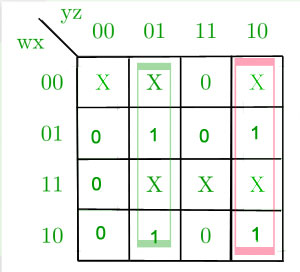K-Map for B-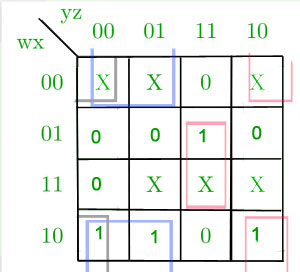K-Map for A-
<img src=”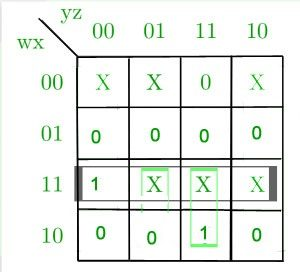Corresponding minimized boolean expressions for Excess-3 code bits –The corresponding digital circuit –
Herecorrespond toandcorrespond to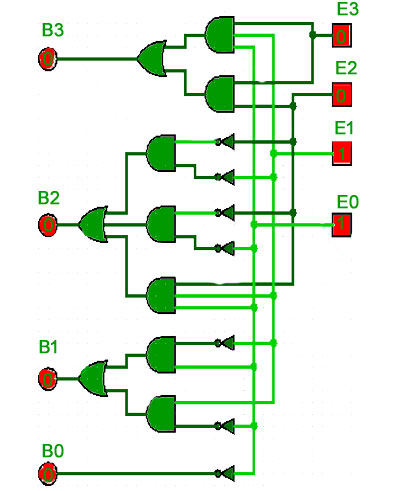This article is contributed by Chirag Manwani. If you like GeeksforGeeks and would like to contribute, you can also write an article using write.geeksforgeeks.org or mail your article to review-team@geeksforgeeks.org. See your article appearing on the GeeksforGeeks main page and help other Geeks.